﻿ Technical Notes > Applications in Electromagnetics > Electrostatics

# Electrostatics

Navigation:  Technical Notes > Applications in Electromagnetics >

# ElectrostaticsPerhaps the most important of all partial differential equations is the simple form

(1.1)It is encountered in virtually all branches of science and engineering, and describes the diffusion of a quantitywith diffusivity k and volume source q.  With k=1 it is referred to as Poisson’s equation,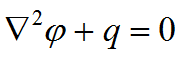.  With k=1 and q=0, it is referred to as Laplace’s equation,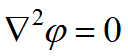.

If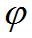is electric potential, k is permittivity and q is charge density, then (1.1) is the electrostatic field equation.

Ifis temperature, k is thermal conductivity and q is heat source, then (1.1) is the heat equation.

If we identify derivatives ofwith fluid velocities,,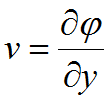then (1.1) is the potential flow equation.

In most cases, we can identifywith the flux of some quantity such as heat, mass or a chemical. (1.1) then says that the variation of the rate of transfer of the relevant quantity is equal to the local source (or sink) of the quantity.

If we integrate the divergence term by parts (or equivalently, apply the divergence theorem), we get

(1.2)That is, the total interior source is equal to the net flow across the outer boundary.

In a FlexPDE script, the equation (1.1) is represented simply as

The boundary flow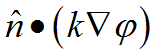is represented in FlexPDE by the Natural boundary condition,